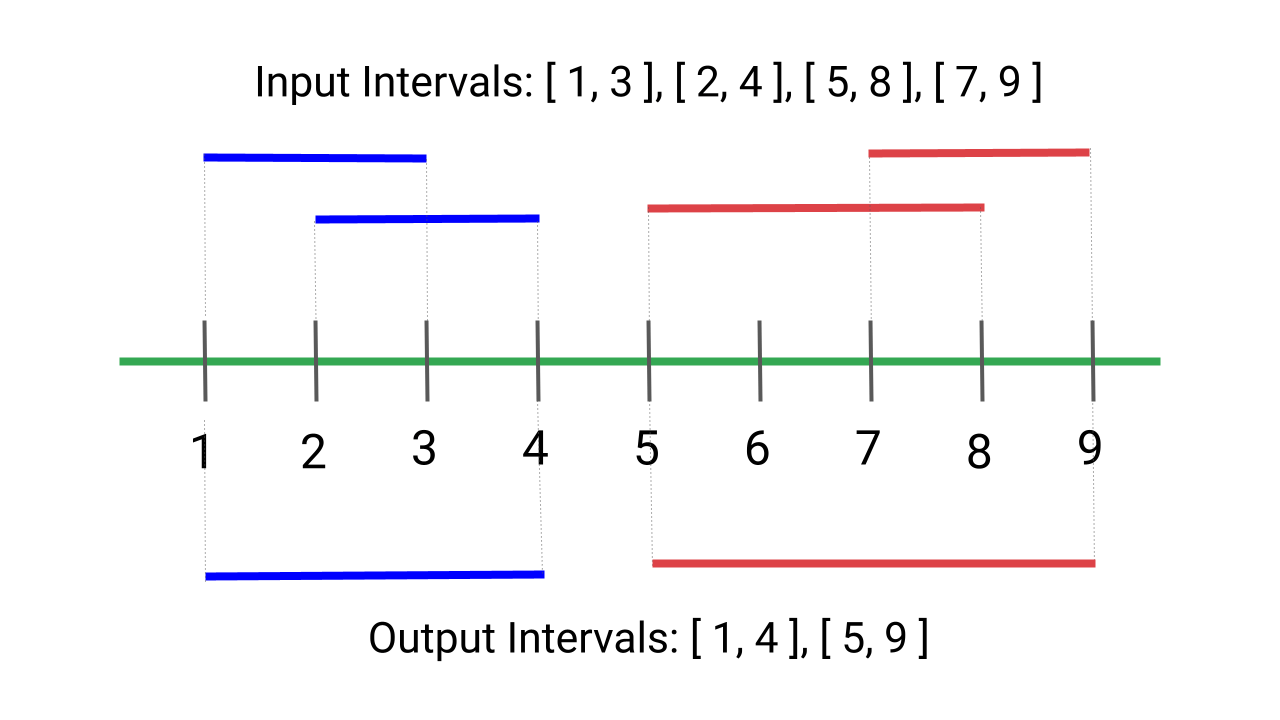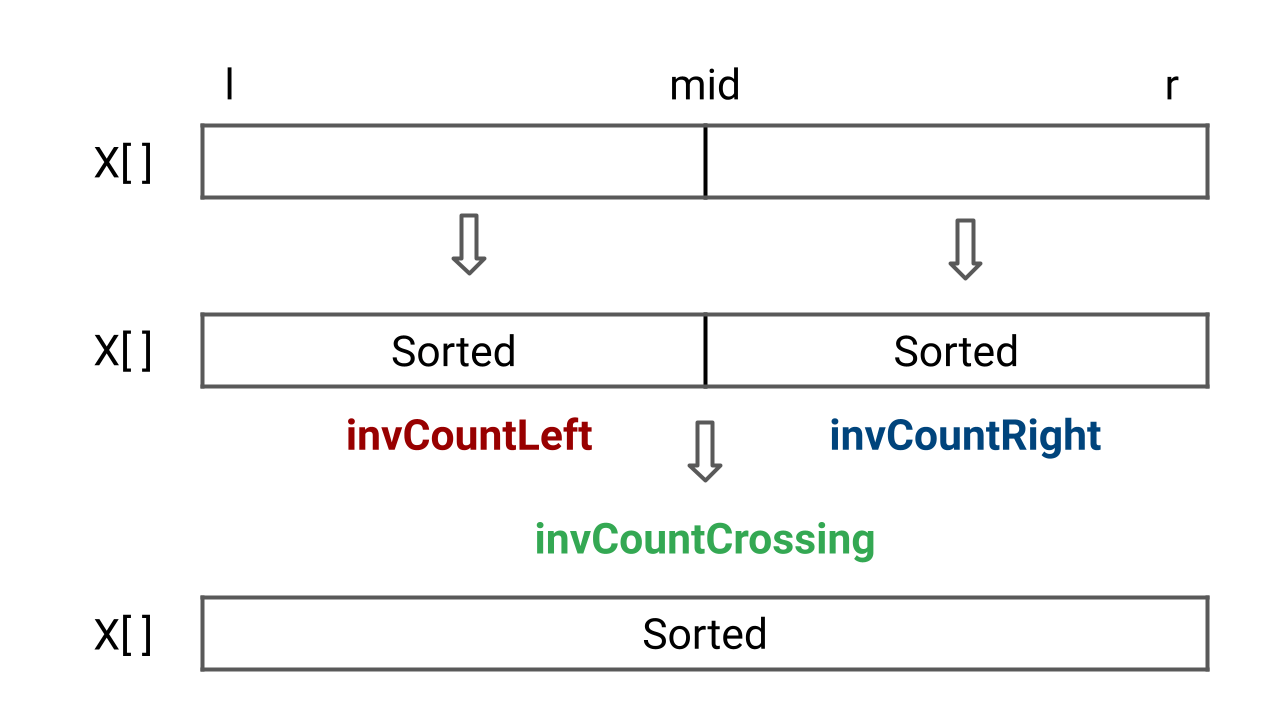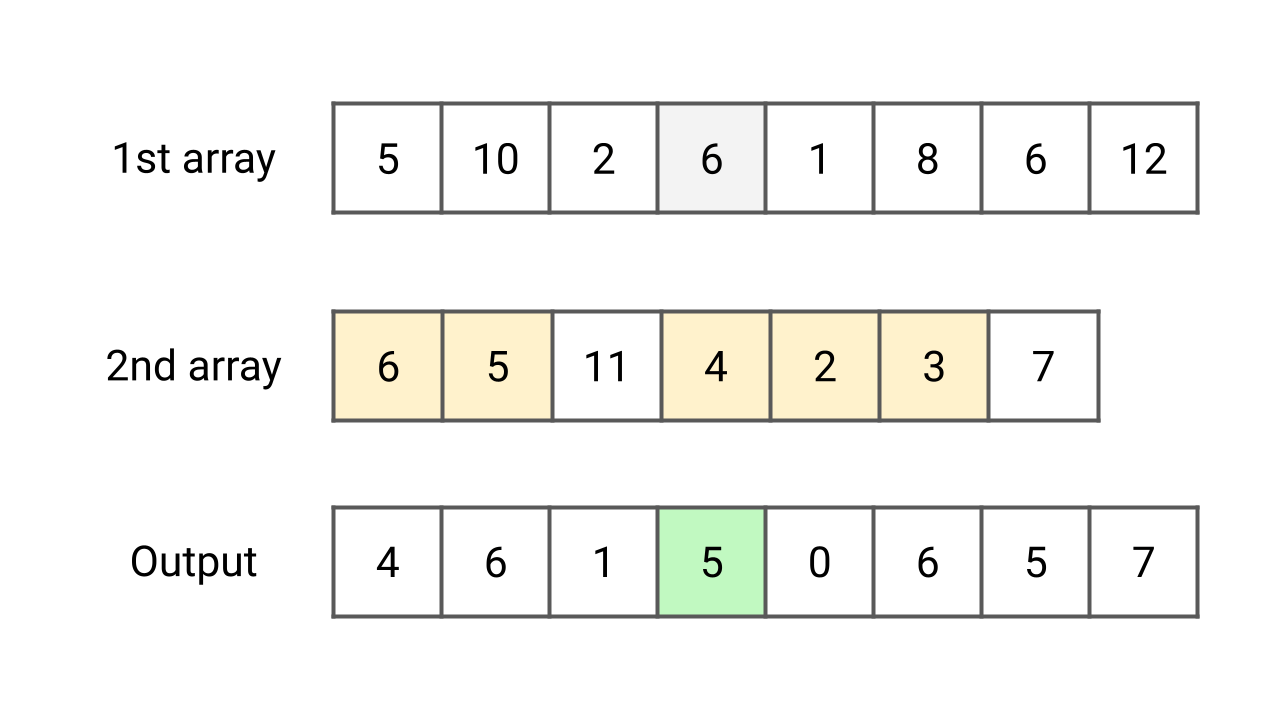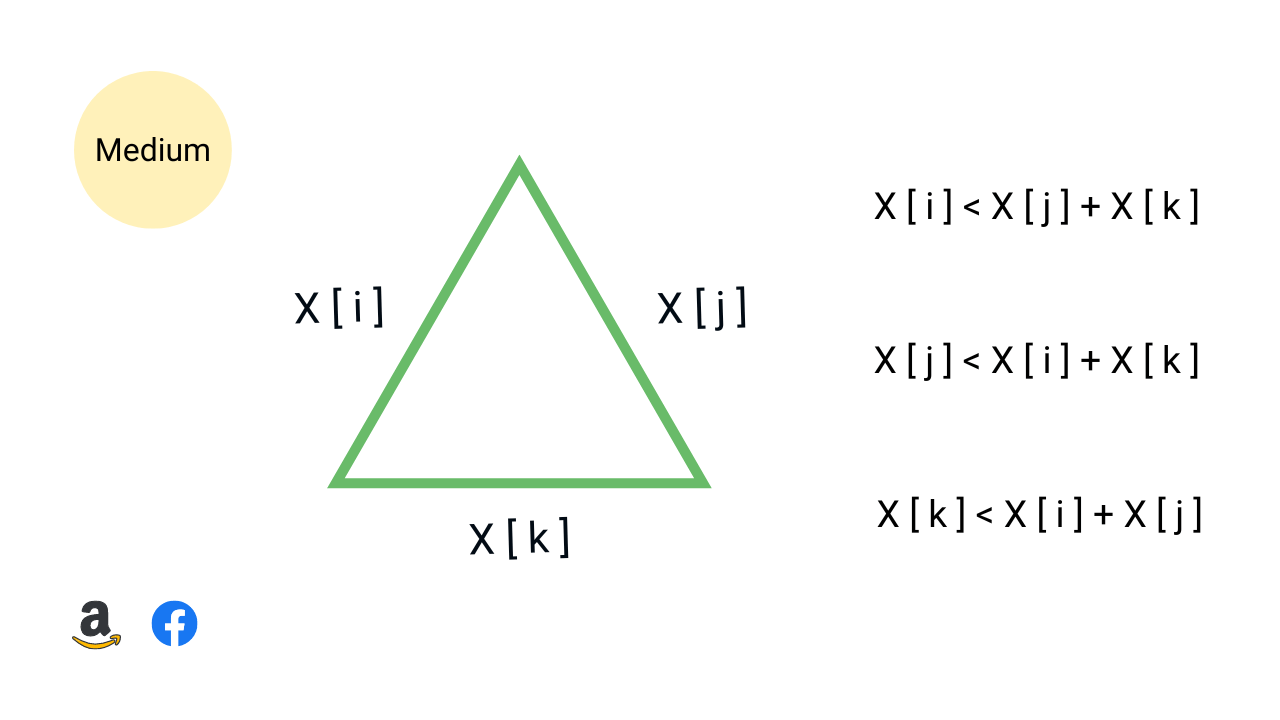# sortingPair sum in an array

Given an array of n integers and a target number, write a program to find whether a pair sum exists in the array or not. In other words, we need to check for a pair of elements in the array that sum exactly to the target value. Assume that all elements are distinct. Note: This is an excellent problem to learn problem solving using two pointers and hash table.Most Frequent Element in an Array

Given an array X[] of size n, write a program to find the most frequent element in the array, i.e. the element which occurs the most number of times. If multiple elements have maximum frequency, return the smallest (assume that at least one element is repeated). Note: This is an excellent problem to learn problem-solving using sorting and hash table.Comparison of Sorting Algorithms

Comparison of sorting algorithms based on different parameters helps us choose an efficient sorting approach. In this blog, we have covered these concepts: 1) What is comparison based sorting? 3) Which sorting is best in terms of time complexity? 3) How to compare sorting algorithms in terms of properties like in-place, stability, etc.?Merge Overlapping Intervals

Given an array of intervals where the start and end points of each interval are provided, write a program to merge all overlapping intervals and return an array of non-overlapping intervals. In other words, the output should be an array of mutually exclusive intervals. Note: This is an excellent problem to learn problem solving using sorting.Count Inversions of an Array

Given an array of n integers, write a program to find the total number of inversion counts. An inversion occurs when there are two elements in the array such that i < j and X[i] > X[j]. Here, the pair (i, j) is called an inversion of X[], and the inversion count represents the count of such inversions present in the array.Each value in 1st array count values less than or equal to in 2nd array

Given two unsorted arrays X[] and Y[] of size m and n. For each element in the first array X[] count the elements in the second array Y[] whose value is less than or equal to it. There can be duplicate elements in the arrays. Note: This is an excellent problem to learn problem solving using sorting and searching.Quicksort Algorithm

Quicksort algorithm is often the best choice for sorting because it works efficiently on average O(nlogn) time complexity. It is also one of the best algorithms to learn divide and conquer approach. In this blog, you will learn: 1) How quick sort works? 2) How to choose a good pivot? 3) Best, worst, and average-case analysis 4) Space complexity and properties of quicksort.Merge Sort Algorithm

Merge sort is one of the fastest comparison-based sorting algorithms, which works on the idea of a divide and conquer approach. The worst and best-case time complexity of the merge sort is O(nlogn), and the space complexity is O(n). It is also one of the best algorithms for sorting linked lists and learning the design and analysis of recursive algorithms.Longest Consecutive Sequence

Given an array of n integers, find the length of the longest consecutive sequence. In other words, we need to find the length of the longest subsequence such that elements in the subsequence are consecutive integers. The consecutive numbers can be in any order. Note: This is an excellent problem to learn problem-solving using sorting and hash table.Count the number of possible triangles

Write a program to count the number of possible triangles that can be formed with three elements from a given unsorted array of positive integers. The three elements, representing the lengths of the sides of a triangle, must satisfy the triangle inequality: the sum of any two sides must be greater than the third side.Counting Sort Algorithm

Counting sort is a stable sorting algorithm that works in O(n) time and space complexity when input are integers in the range 0 to k and k = O(n). Instead of comparison, counting sort uses array indexing to determine position of elements. For each element x, it counts values less than x and places x directly into its correct position in the sorted array.Sort a Stack

Given a stack, write a program to sort a stack in ascending order. We are not allowed to make assumptions about how the stack is implemented. The only functions to be used are push(s, x), pop(s), top(s), isEmpty(s). In this blog, we have discussed two approaches: 1) Sorting stack using temporary stack 2) Sorting stack using recursion.Maximum Index

Given an array X[] of integers, find the maximum parity index. The parity index is the maximum index difference between two elements (X[i], X[j]) such that, j > i and X[j] > X[i]. In other words, we need to find maximum j - i such that j > i and X[j] > X[i]. Note: This is an excellent problem to learn problem solving using sorting and two pointers approachesArray Subset of Another Array

Given two unsorted arrays of size m and n, find whether one array is a subset of another array or not. An array Y[] will be a subset of another array X[] if each element of Y[] is present in X[]. Assume that there are no repeated elements in both arrays and n <= m. Note: This is an excellent problem to learn problem solving using various approaches.Find Triplets with Zero Sum

Given an array X[] of n distinct elements, write a program to find all the unique triplets in the array whose sum is equal to zero. For example, if triplets with zero sum in the array are (X[i], X[j], X[k]), then X[i] + X[j] + X[k] = 0. Hint: This is an excellent problem to learn problem-solving and optimization using hashing and two pointers approach.First Missing Positive

Given an array that includes both positive and negative numbers, write a program to find the first missing positive integer. This is one of the best problems for learning step-by-step time complexity optimization using various approaches. An in-place hashing solution uses the same input array to process values and generate output.Sort an Array of 0s, 1s and 2s (Dutch National Flag Problem)

Given an array consisting of 0s, 1s, and 2s, write a program to sort this array of 0, 1, and 2 in ascending order. We need to sort the array in O(n) time complexity without using sorting algorithms or extra space. Note: This is a variation of the Dutch national flag problem and an excellent problem to learn problem solving using three pointers.Bubble Sort, Selection Sort and Insertion Sort Algorithm

Learn the design, implementation, analysis, and comparison of bubble sort, selection sort, and insertion sort. In data structures and algorithms, these are some of the fundamental sorting algorithms to learn problem-solving using an incremental approach with the help of nested loops. All of them have the same worst-case and average-case time complexity.Wave Array Problem

Given an unsorted array of n integers, write a program to sort array into a wave array. There can be many possible waveforms, but we need to return any one of them. An array A[] is sorted in wave arrangement if A >= A <= A >= A <= A >= ....and so on. Note: This is an excellent problem to learn problem-solving using a single loop.Check if two arrays are equal or not

Given two integer arrays X[] and Y[], write a program to check if arrays are equal or not. Two arrays are equal if they have the same elements in any order. If there are repeated elements, then counts of repeated elements must also be the same for both arrays. Note: This is an excellent problem to learn problem solving using a hash table.Lower Bound of Comparison Based Sorting

Sorting algorithms like merge sort, quicksort, insertion sort, heap sort, etc., determine sorted order based on comparison operations. We call such algorithms comparison sort. The tightest lower bound on the number of comparisons performed by comparison based sorting is O(nlogn). In this blog, we have used a decision tree model to prove this.Sort Characters by Frequency

Given a string S[], write a program to sort string S in decreasing order based on the frequency of characters. The frequency of a character is the number of times it appears in the string. If two characters have the same frequency, whichever occurs earliest in S, must come first. In other words, frequency based sorting must be stable.We have given the head of a singly linked list, write a program to sort the list using insertion sort, and return the head of the sorted linked list. To implement this algorithm, we need to understand the idea of insertion sort on array. At each step of the iteration, insertion sort adds one element to the sorted output and grows the partially sorted list size by 1.Why do We Need Sorting Algorithms?

Why sorting algorithms are important in data structure? There are various reasons: 1) Sorting helps us to learn both iterative and recursive problem-solving approaches, 2) Sorting is one of the best ideas for learning time complexity analysis, code optimization techniques, etc. 3) We can solve several coding problems efficiently by sorting the input data.